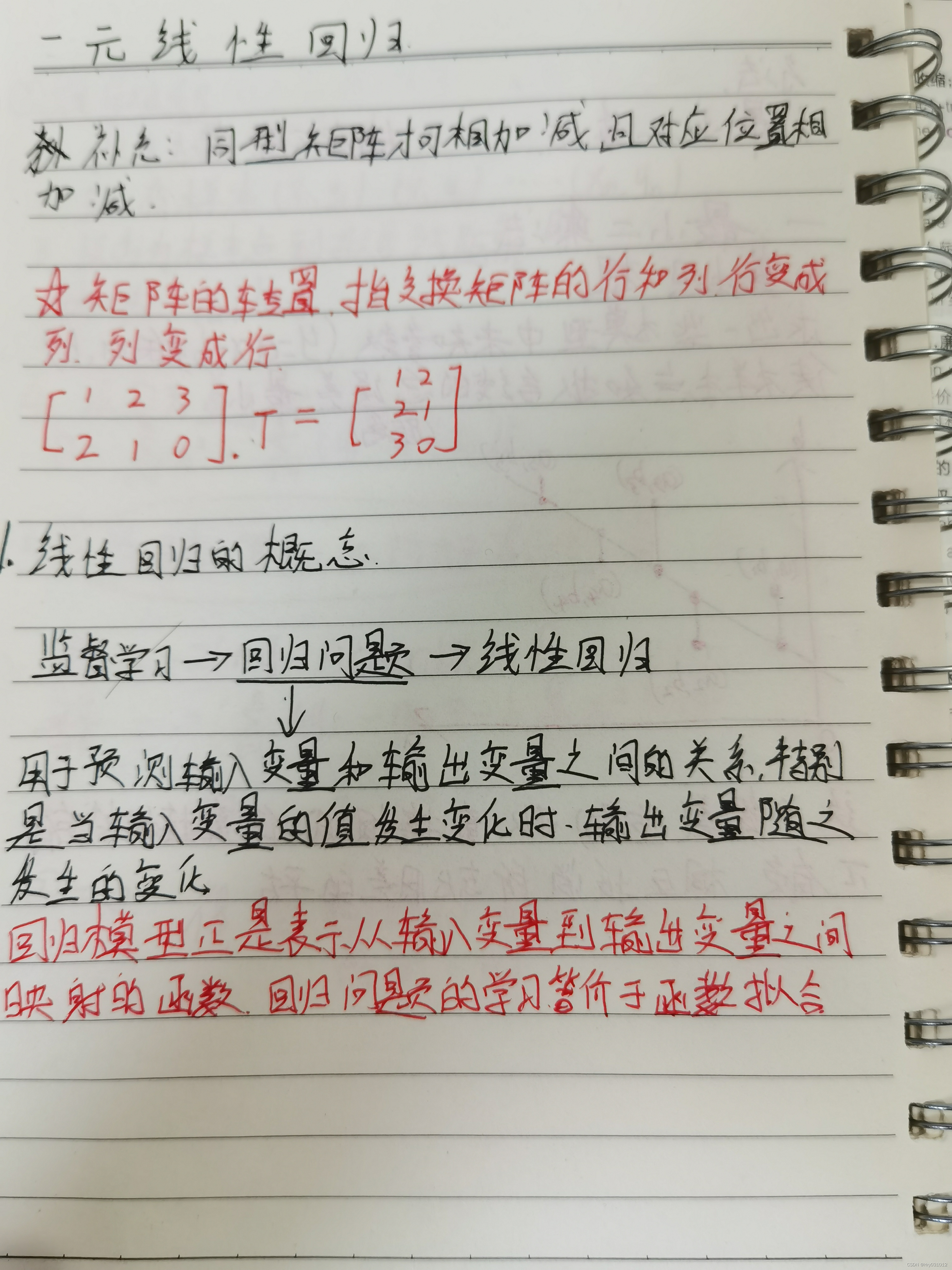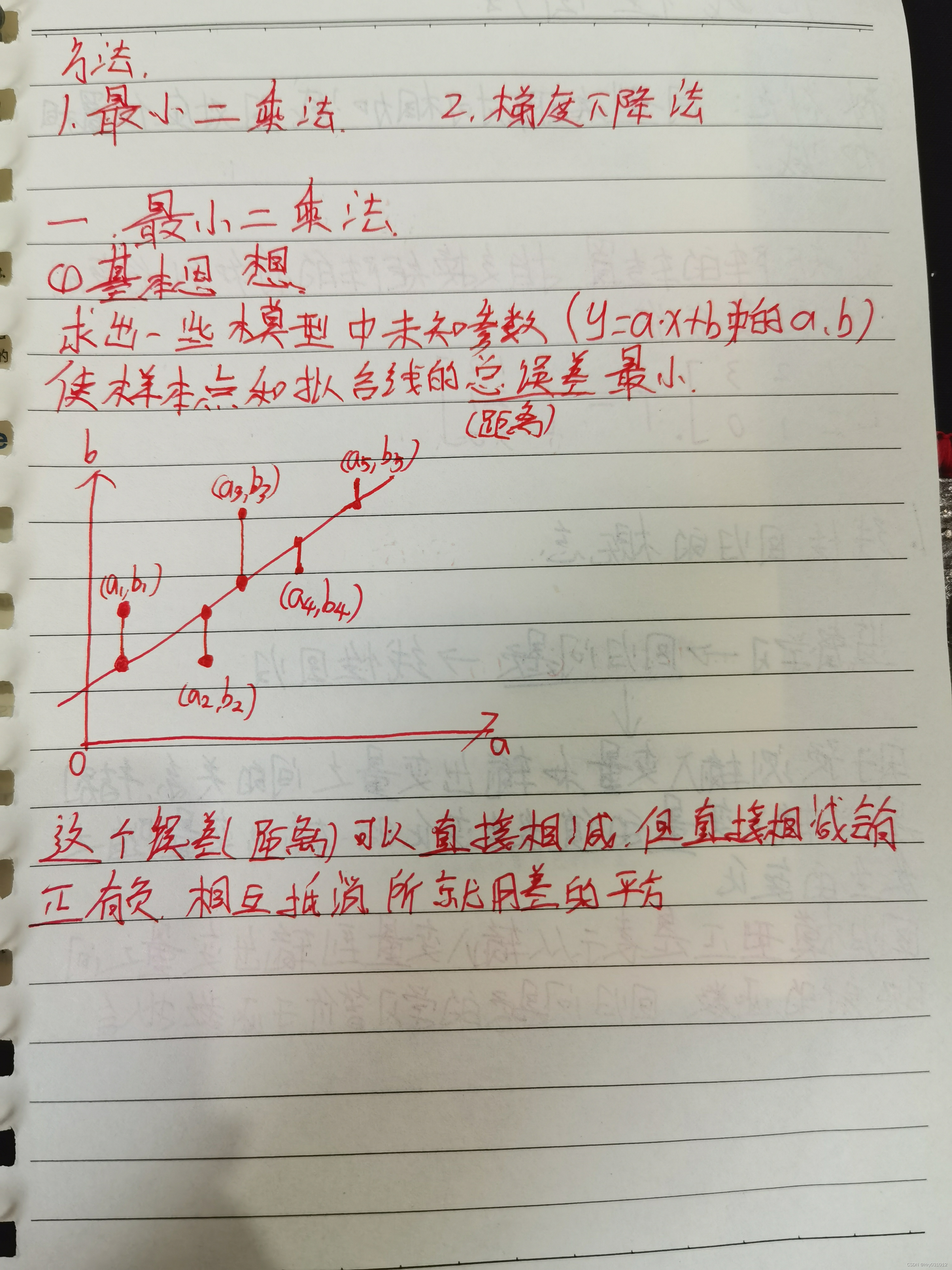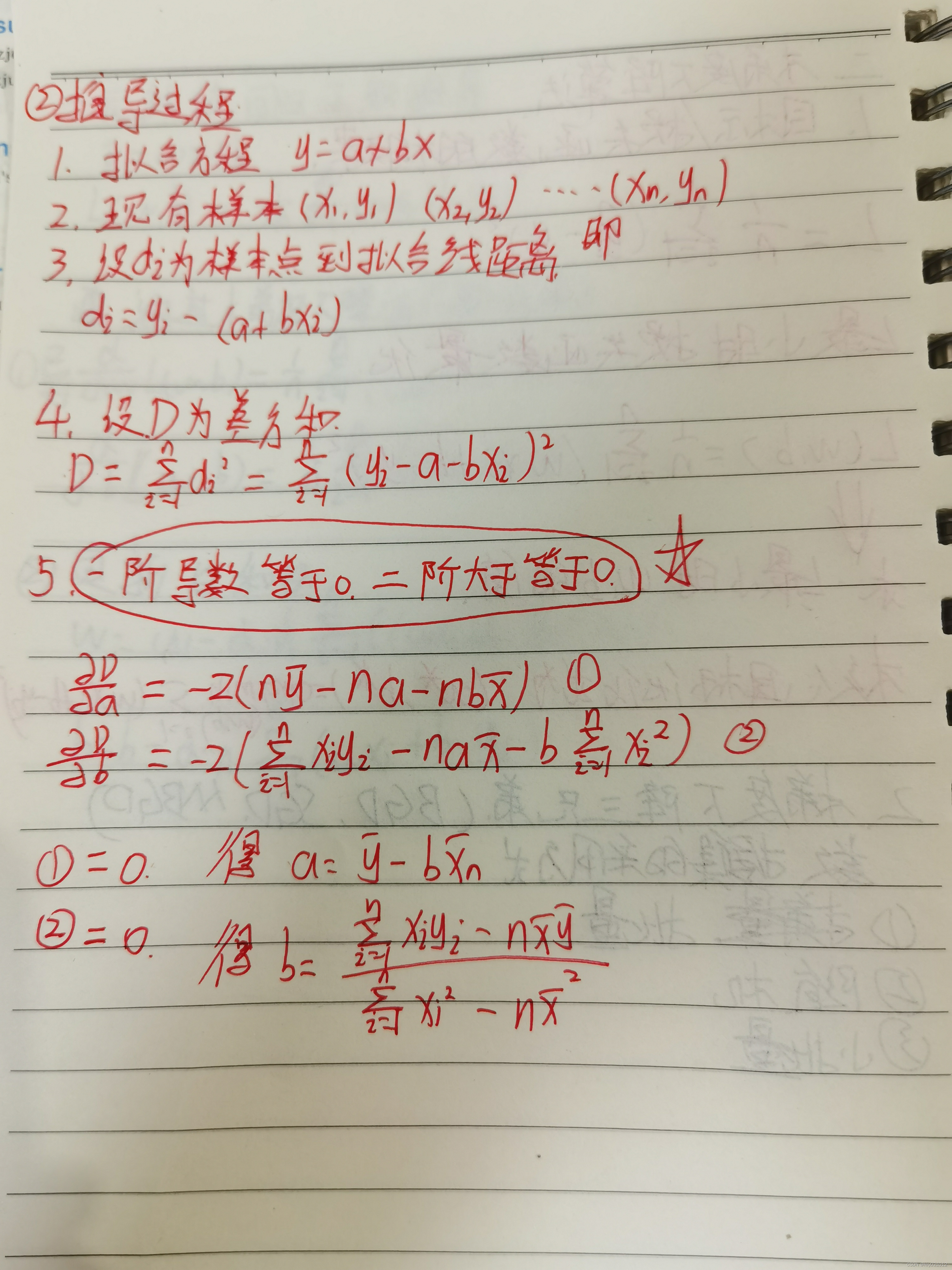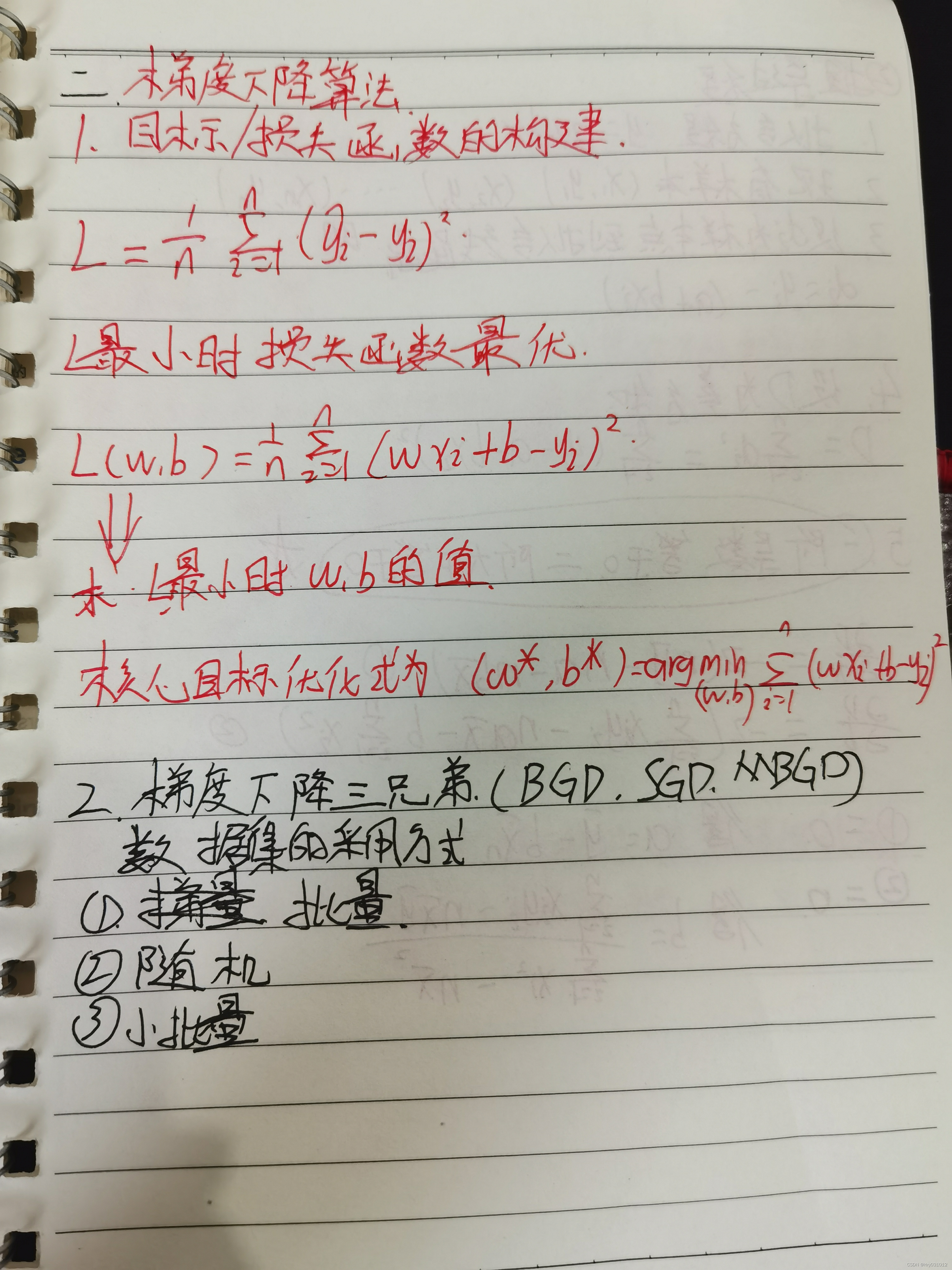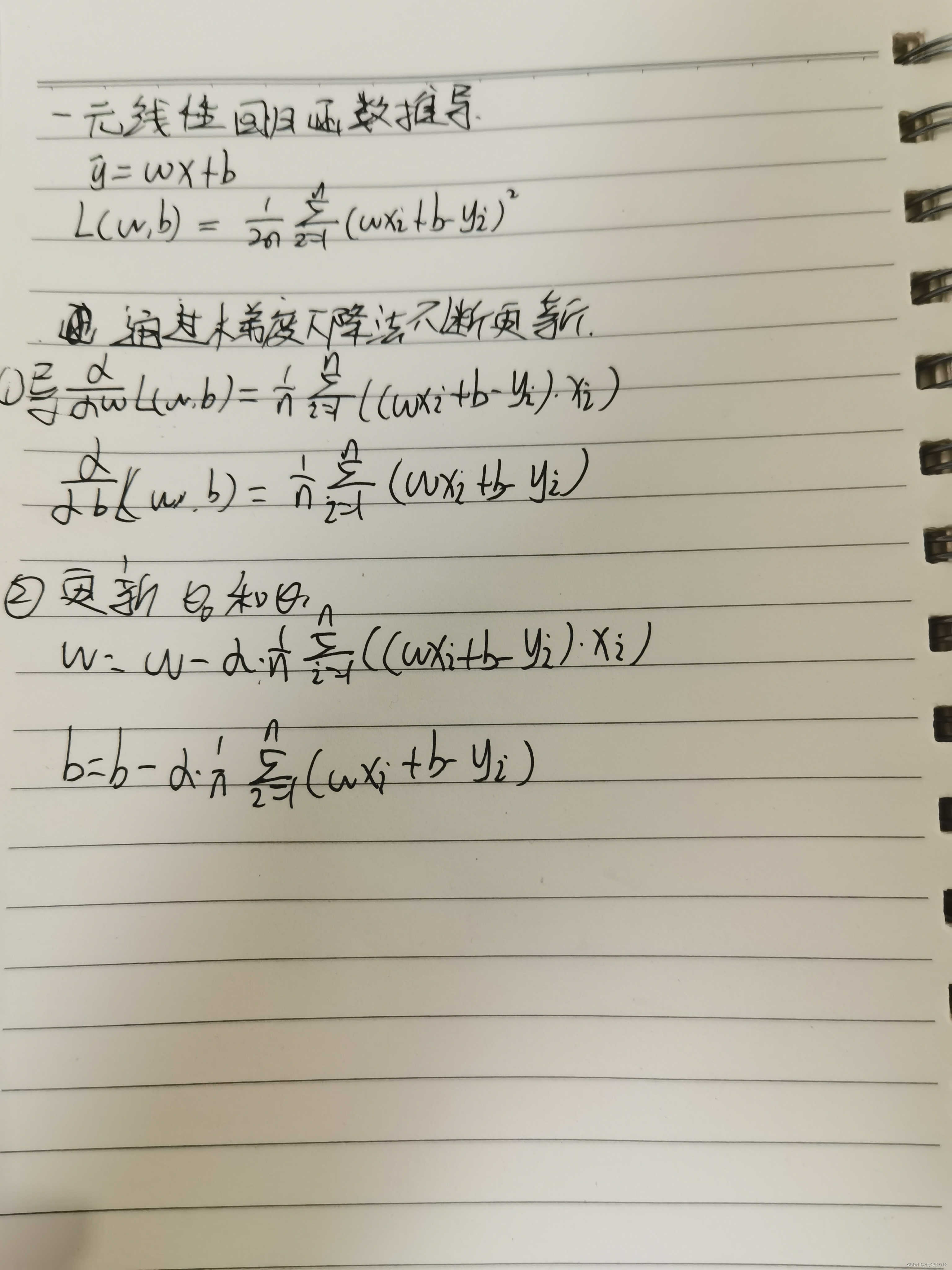# （补充）矩阵的转置

矩阵的转置是指交换矩阵的行和列，行变成列，列变成行

对于[ 123210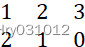].T  =  [122130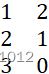]

# 1.线性回归的概念

监督学习->回归问题->线性回归

用于预测输入变量和输出变量之间的关系，特别是当输入变量的值发生变化时，输出变量的值随之发生的变化。回归模型正是表示从输入变量到输出变量之间映射的函数回归问题的学习等价于函数拟合

# 2.线性回归的分类

如果研究的线性代数只包含一个自变量和一个因变量且二者的关系可以通过一条直线近似的刻画时，这种回归就称为一元线性回归。

如果回归分析中涉及到两个及以上的自变量，且自变量与因变量是线性关系，就称为多元线性回归。

# 3.最小二乘法

求出一些模型中未知参数（例如：y=a*x+b中的参数ab使得样本点和拟合线的总误差（距离）最小最直观的感受如下图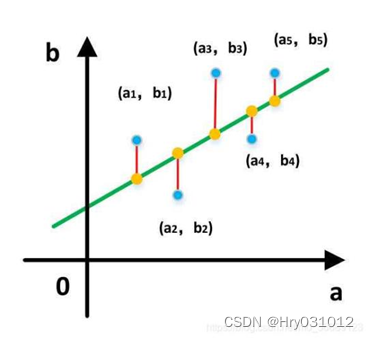而这个误差（距离）可以直接相减，但是直接相减会有正有负，相互抵消了，所以就用差的平方

推导过程：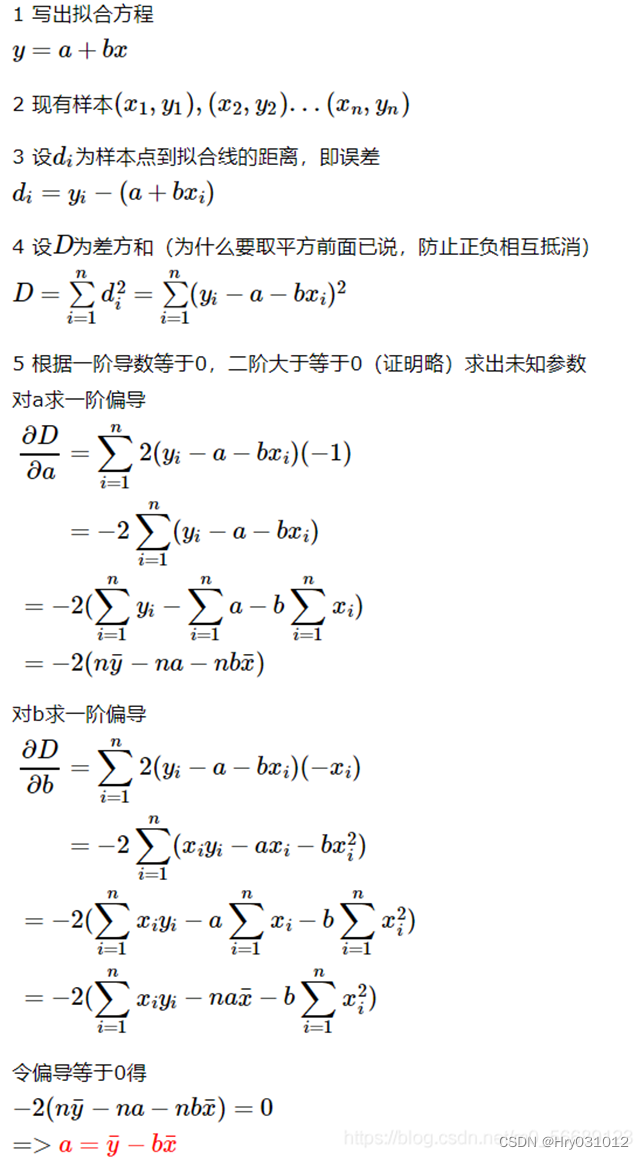最小二乘法代码 ：

``	import numpy as np	from matplotlib import pylab as pl	#Defining training data	x = np.array([1,3,2,1,3])	y = np.array([14,24,18,17,27])	# The regression equation takes the function	def fit(x,y):	    if len(x) != len(y):	        return	    numerator = 0.0	    denominator = 0.0	    x_mean = np.mean(x)	    y_mean = np.mean(y)	    for i in range(len(x)):	        numerator += (x[i]-x_mean)*(y[i]-y_mean)	        denominator += np.square((x[i]-x_mean))	    print('numerator:',numerator,'denominator:',denominator)	    b0 = numerator/denominator	    b1 = y_mean - b0*x_mean	    return b0,b1	# Define prediction function	def predit(x,b0,b1):	    return b0*x + b1	# Find the regression equation	b0,b1 = fit(x,y)	print('Line is:y = %2.0fx + %2.0f'%(b0,b1))	# prediction	x_test = np.array([0.5,1.5,2.5,3,4])	y_test = np.zeros((1,len(x_test)))	for i in range(len(x_test)):	    y_test[i] = predit(x_test[i],b0,b1)	# Drawing figure	xx = np.linspace(0, 5)	yy = b0*xx + b1	pl.plot(xx,yy,'k-')	pl.scatter(x,y,cmap=pl.cm.Paired)	pl.scatter(x_test,y_test,cmap=pl.cm.Paired)	pl.show()``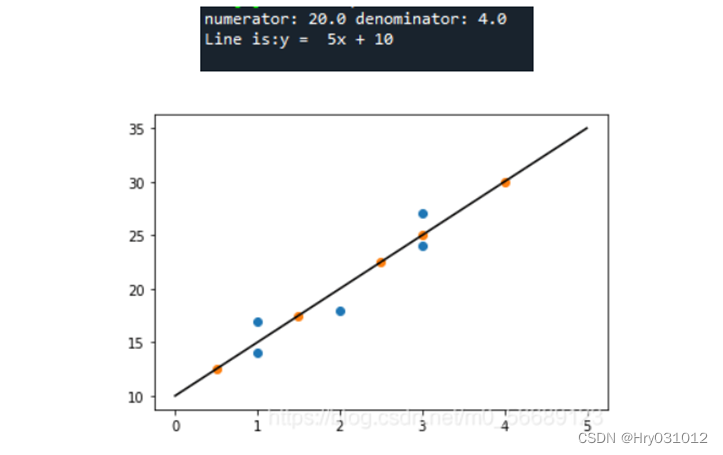# 4.梯度下降算法

1目标/损失函数的构建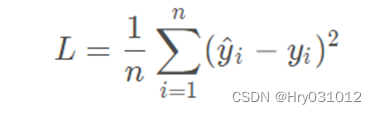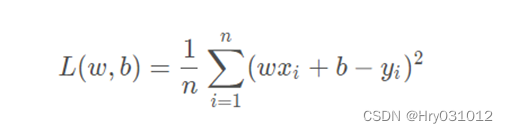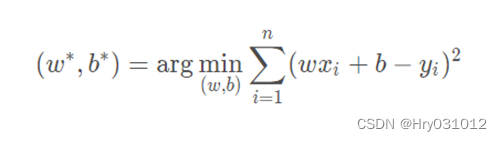2 梯度下降三兄弟（BGDSGD MBGD

3 梯度下降法的一般步骤

①首先设定一个较小的正数 α , ε;

②求当前位置出处的各个偏导数：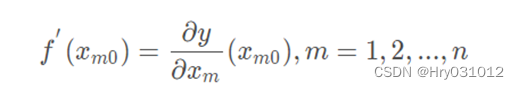③修改当前函数的参数值，公式如下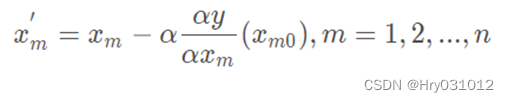④如果参数变化量小于 ，退出；否则返回第2步。

4 一元线性回归函数推导过程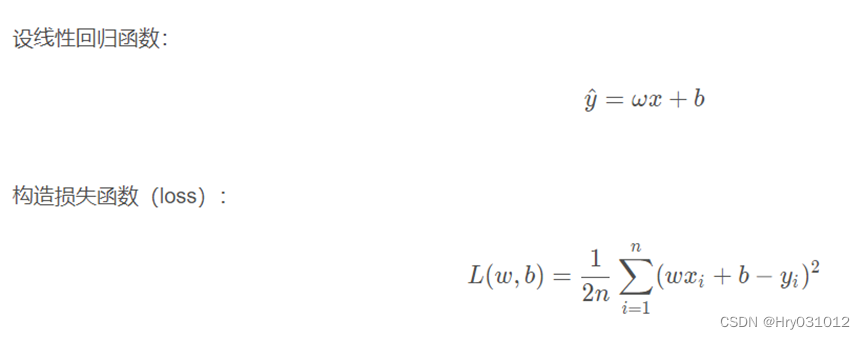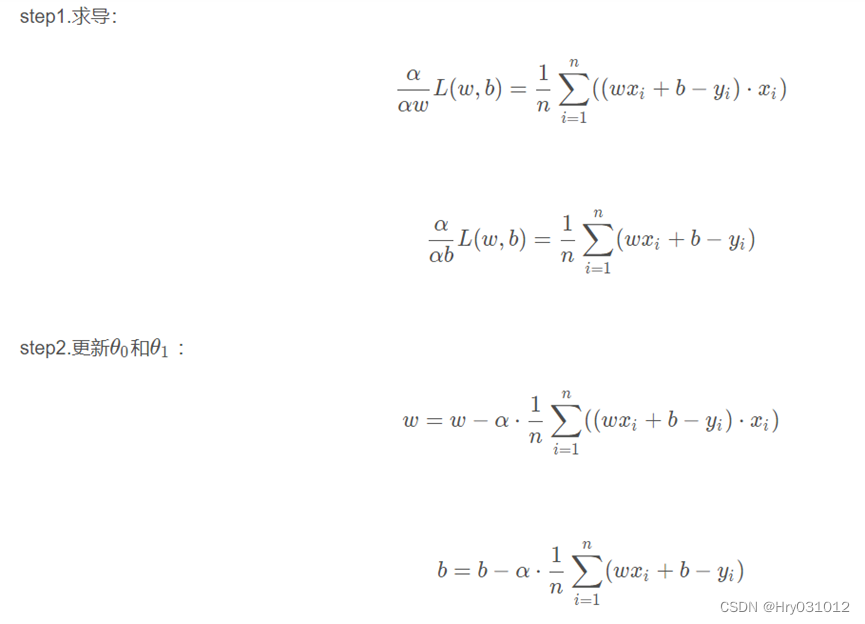5(实例)波士顿房价预测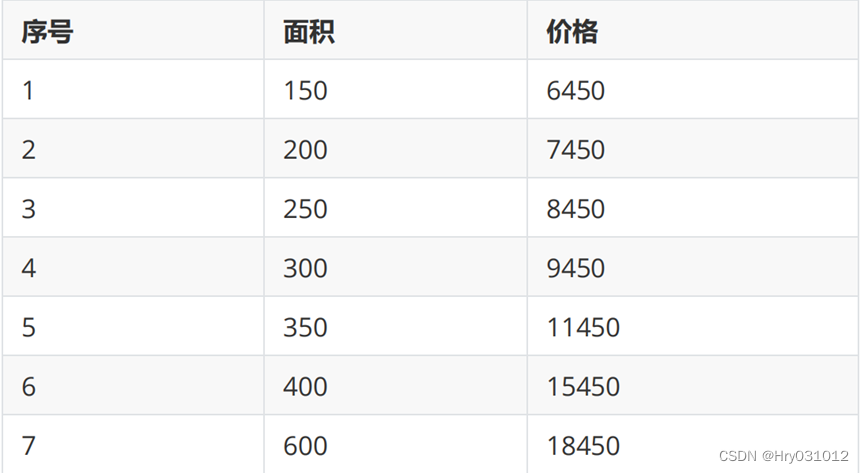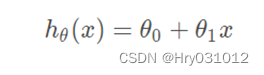Python代码：

``	#房屋价格与面积	#序号：1     2    3    4     5     6     7	#面积：150  200  250  300   350   400   600  	#价格：6450 7450 8450 9450 11450 15450 18450 	import matplotlib.pyplot as plt	import matplotlib	from math import pow	import random	x0 = [150,200,250,300,350,400,600]	y0 = [6450,7450,8450,9450,11450,15450,18450]	#为了方便计算，将所有数据缩小 100 倍	x = [1.50,2.00,2.50,3.00,3.50,4.00,6.00]	y = [64.50,74.50,84.50,94.50,114.50,154.50,184.50]	#线性回归函数为 y=theta0+theta1*x	#损失函数 J (θ)=(1/(2*m))*pow((theta0+theta1*x[i]-y[i]),2)	#参数定义	theta0 = 0.1#对 theata0 赋值	theta1 = 0.1#对 theata1 赋值	alpha = 0.1#学习率	m = len(x)	count0 = 0	theta0_list = []	theta1_list = []	#1.使用批量梯度下降法	for num in range(10000):	    count0 += 1	    diss = 0   #误差	    deriv0 = 0 #对 theata0 导数	    deriv1 = 0 #对 theata1 导数	    #求导	    for i in range(m):	        deriv0 += (theta0+theta1*x[i]-y[i])/m#对每一项测试数据求导再求和取平均值	        deriv1 += ((theta0+theta1*x[i]-y[i])/m)*x[i]	    #更新 theta0 和 theta1	    theta0 = theta0 - alpha*deriv0 	    theta1 = theta1 - alpha*deriv1	    #求损失函数 J (θ)	    for i in range(m):	        diss = diss + (1/(2*m))*pow((theta0+theta1*x[i]-y[i]),2)	            	    theta0_list.append(theta0*100)	    theta1_list.append(theta1)	    #如果误差已经很小，则退出循环	    if diss <= 0.001:	        break	theta0 = theta0*100#前面所有数据缩小了 100 倍，所以求出的 theta0 需要放大 100 倍，theta1 不用变	#2.使用随机梯度下降法	theta2 = 0.1#对 theata2 赋值	theta3 = 0.1#对 theata3 赋值	count1 = 0	theta2_list = []	theta3_list = []	for num in range(10000):	    count1 += 1	    diss = 0   #误差	    deriv2 = 0 #对 theata2 导数	    deriv3 = 0 #对 theata3 导数	    #求导	    for i in range(m):	        deriv2 += (theta2+theta3*x[i]-y[i])/m	        deriv3 += ((theta2+theta3*x[i]-y[i])/m)*x[i]	    #更新 theta0 和 theta1	    for i in range(m):	        theta2 = theta2 - alpha*((theta2+theta3*x[i]-y[i])/m) 	        theta3 = theta3 - alpha*((theta2+theta3*x[i]-y[i])/m)*x[i]	    #求损失函数 J (θ)	    rand_i = random.randrange(0,m)	    diss = diss + (1/(2*m))*pow((theta2+theta3*x[rand_i]-y[rand_i]),2)	    	    theta2_list.append(theta2*100)	    theta3_list.append(theta3)	    #如果误差已经很小，则退出循环	    if diss <= 0.001:	        break	theta2 = theta2*100	print("批量梯度下降最终得到theta0={}，theta1={}".format(theta0,theta1))	print("           得到的回归函数是：y={}+{}*x".format(theta0,theta1))	print("随机梯度下降最终得到theta0={}，theta1={}".format(theta2,theta3))	print("           得到的回归函数是：y={}+{}*x".format(theta2,theta3))	#画原始数据图和函数图	matplotlib.rcParams['font.sans-serif'] = ['SimHei']	plt.plot(x0,y0,'bo',label='数据',color='black')	plt.plot(x0,[theta0+theta1*x for x in x0],label='批量梯度下降',color='red')	plt.plot(x0,[theta2+theta3*x for x in x0],label='随机梯度下降',color='blue')	plt.xlabel('x（面积）')	plt.ylabel('y（价格）')	plt.legend()	plt.show()	plt.scatter(range(count0),theta0_list,s=1)	plt.scatter(range(count0),theta1_list,s=1)	plt.xlabel('上方为theta0，下方为theta1')	plt.show()	plt.scatter(range(count1),theta2_list,s=3)	plt.scatter(range(count1),theta3_list,s=3)	plt.xlabel('上方为theta0，下方为theta1')	plt.show()``

结果显示：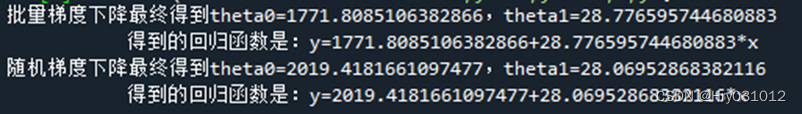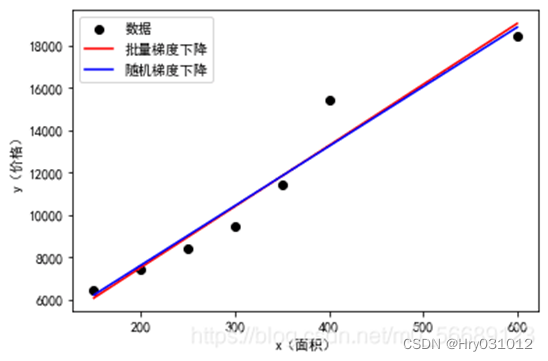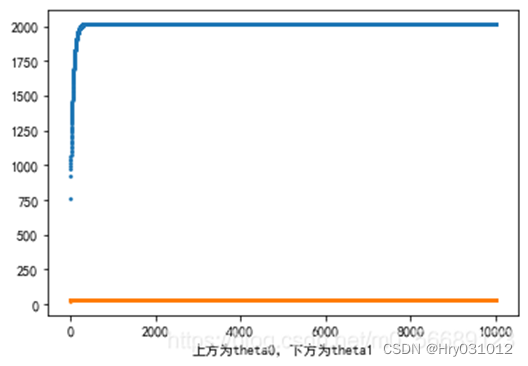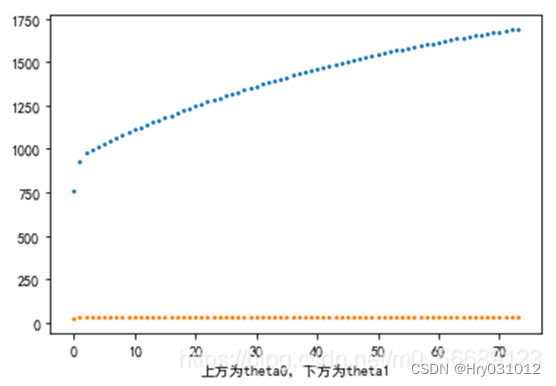# 我的笔记：